# Binary Number System and Codes

Dec. 5, 2021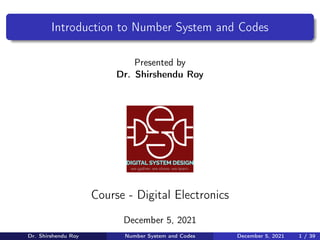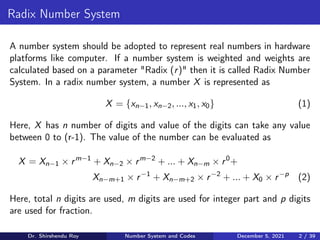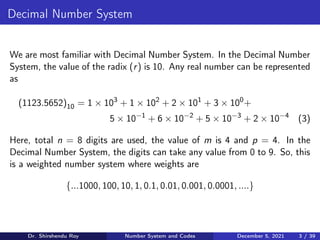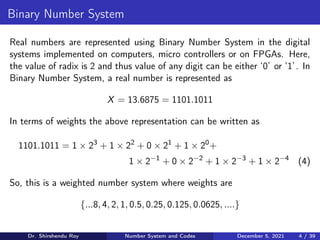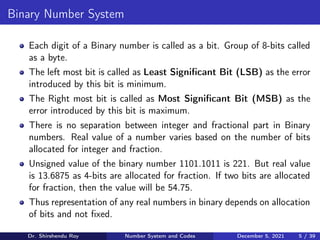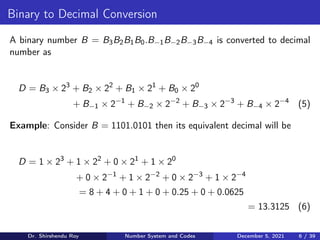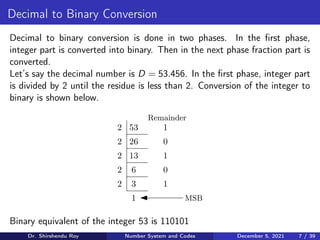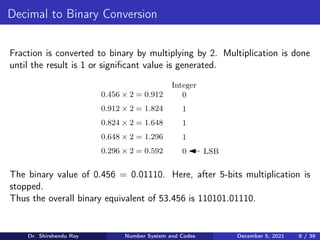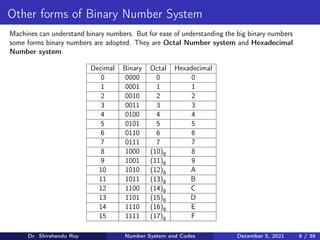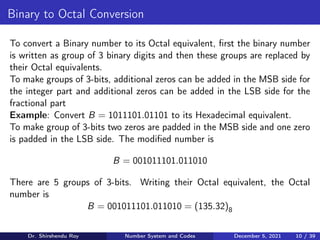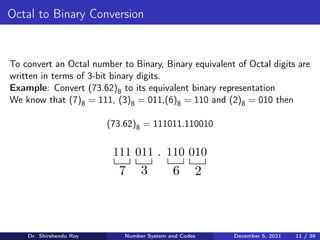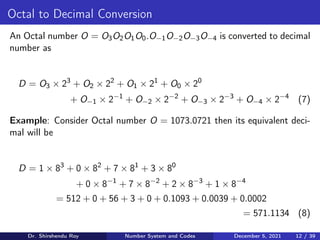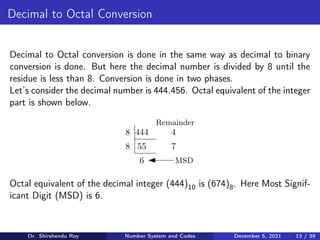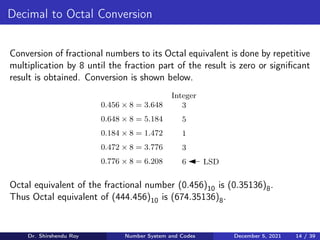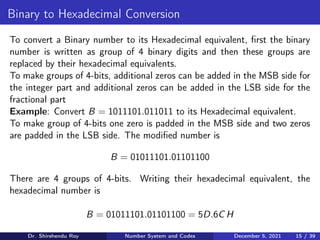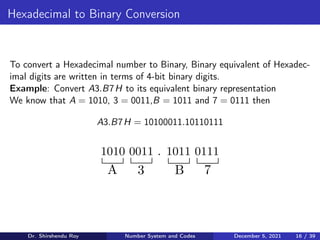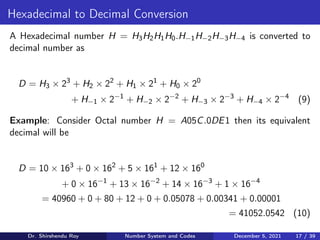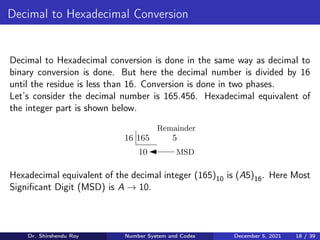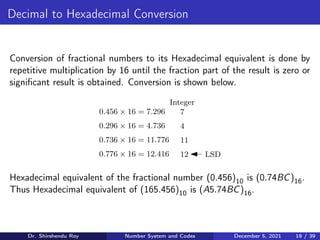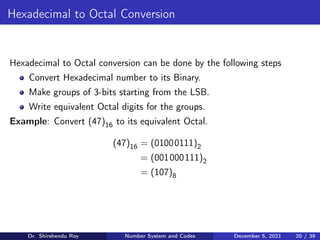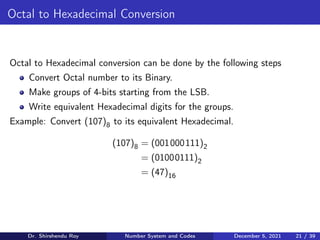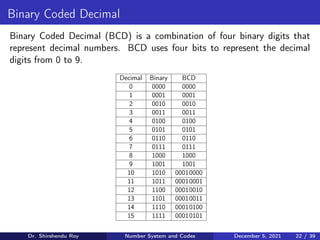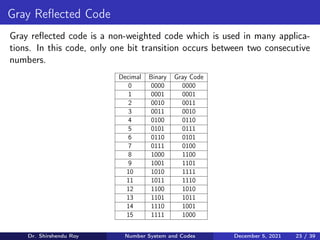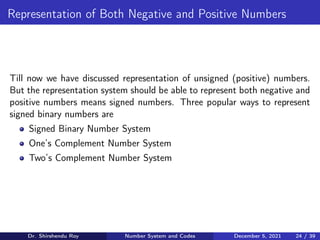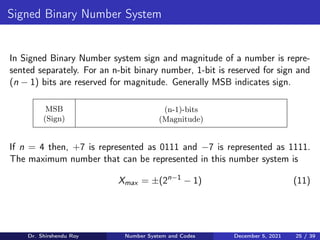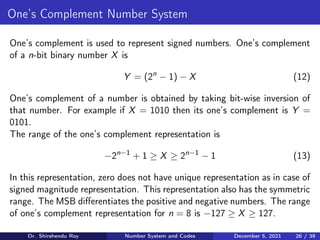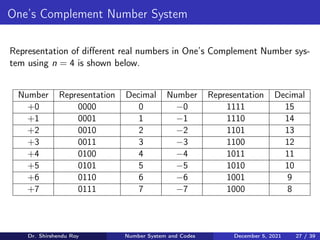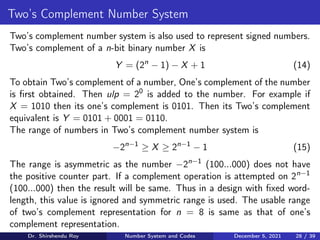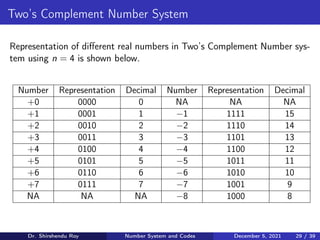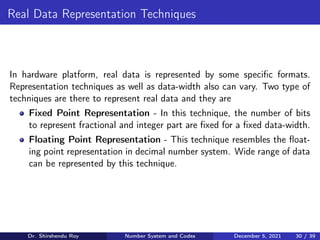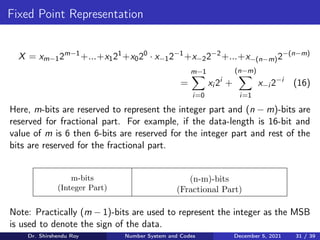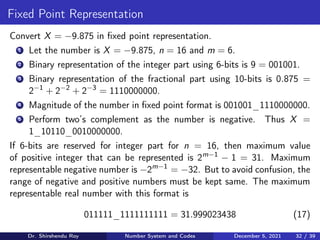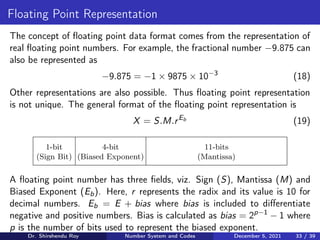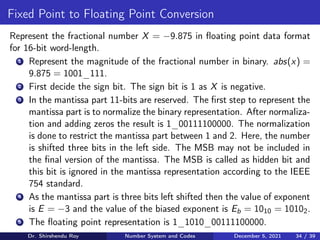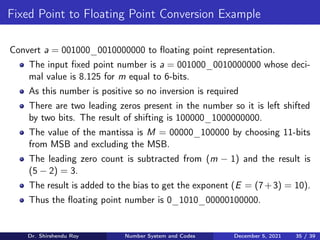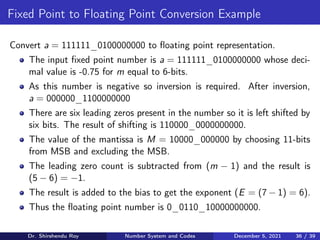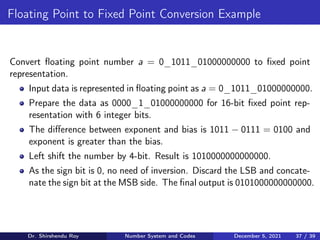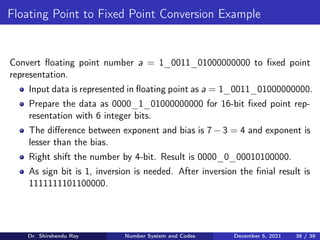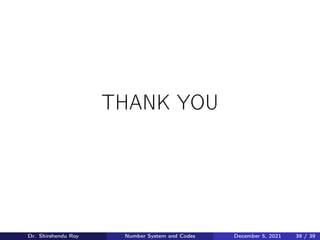1 of 39

### Binary Number System and Codes

• 1. Introduction to Number System and Codes Presented by Dr. Shirshendu Roy Course - Digital Electronics December 5, 2021 Dr. Shirshendu Roy Number System and Codes December 5, 2021 1 / 39
• 2. Radix Number System A number system should be adopted to represent real numbers in hardware platforms like computer. If a number system is weighted and weights are calculated based on a parameter "Radix (r)" then it is called Radix Number System. In a radix number system, a number X is represented as X = {xn−1, xn−2, ..., x1, x0} (1) Here, X has n number of digits and value of the digits can take any value between 0 to (r-1). The value of the number can be evaluated as X = Xn−1 × rm−1 + Xn−2 × rm−2 + ... + Xn−m × r0 + Xn−m+1 × r−1 + Xn−m+2 × r−2 + ... + X0 × r−p (2) Here, total n digits are used, m digits are used for integer part and p digits are used for fraction. Dr. Shirshendu Roy Number System and Codes December 5, 2021 2 / 39
• 3. Decimal Number System We are most familiar with Decimal Number System. In the Decimal Number System, the value of the radix (r) is 10. Any real number can be represented as (1123.5652)10 = 1 × 103 + 1 × 102 + 2 × 101 + 3 × 100 + 5 × 10−1 + 6 × 10−2 + 5 × 10−3 + 2 × 10−4 (3) Here, total n = 8 digits are used, the value of m is 4 and p = 4. In the Decimal Number System, the digits can take any value from 0 to 9. So, this is a weighted number system where weights are {...1000, 100, 10, 1, 0.1, 0.01, 0.001, 0.0001, ....} Dr. Shirshendu Roy Number System and Codes December 5, 2021 3 / 39
• 4. Binary Number System Real numbers are represented using Binary Number System in the digital systems implemented on computers, micro controllers or on FPGAs. Here, the value of radix is 2 and thus value of any digit can be either ‘0’ or ‘1’. In Binary Number System, a real number is represented as X = 13.6875 = 1101.1011 In terms of weights the above representation can be written as 1101.1011 = 1 × 23 + 1 × 22 + 0 × 21 + 1 × 20 + 1 × 2−1 + 0 × 2−2 + 1 × 2−3 + 1 × 2−4 (4) So, this is a weighted number system where weights are {...8, 4, 2, 1, 0.5, 0.25, 0.125, 0.0625, ....} Dr. Shirshendu Roy Number System and Codes December 5, 2021 4 / 39
• 5. Binary Number System Each digit of a Binary number is called as a bit. Group of 8-bits called as a byte. The left most bit is called as Least Significant Bit (LSB) as the error introduced by this bit is minimum. The Right most bit is called as Most Significant Bit (MSB) as the error introduced by this bit is maximum. There is no separation between integer and fractional part in Binary numbers. Real value of a number varies based on the number of bits allocated for integer and fraction. Unsigned value of the binary number 1101.1011 is 221. But real value is 13.6875 as 4-bits are allocated for fraction. If two bits are allocated for fraction, then the value will be 54.75. Thus representation of any real numbers in binary depends on allocation of bits and not fixed. Dr. Shirshendu Roy Number System and Codes December 5, 2021 5 / 39
• 6. Binary to Decimal Conversion A binary number B = B3B2B1B0.B−1B−2B−3B−4 is converted to decimal number as D = B3 × 23 + B2 × 22 + B1 × 21 + B0 × 20 + B−1 × 2−1 + B−2 × 2−2 + B−3 × 2−3 + B−4 × 2−4 (5) Example: Consider B = 1101.0101 then its equivalent decimal will be D = 1 × 23 + 1 × 22 + 0 × 21 + 1 × 20 + 0 × 2−1 + 1 × 2−2 + 0 × 2−3 + 1 × 2−4 = 8 + 4 + 0 + 1 + 0 + 0.25 + 0 + 0.0625 = 13.3125 (6) Dr. Shirshendu Roy Number System and Codes December 5, 2021 6 / 39
• 7. Decimal to Binary Conversion Decimal to binary conversion is done in two phases. In the first phase, integer part is converted into binary. Then in the next phase fraction part is converted. Let’s say the decimal number is D = 53.456. In the first phase, integer part is divided by 2 until the residue is less than 2. Conversion of the integer to binary is shown below. 53 2 Remainder 1 2 26 2 13 2 6 2 3 1 0 1 0 1 MSB Binary equivalent of the integer 53 is 110101 Dr. Shirshendu Roy Number System and Codes December 5, 2021 7 / 39
• 8. Decimal to Binary Conversion Fraction is converted to binary by multiplying by 2. Multiplication is done until the result is 1 or significant value is generated. 0.456 × 2 = 0.912 0.912 × 2 = 1.824 Integer 0 1 0.824 × 2 = 1.648 1 0.648 × 2 = 1.296 1 0.296 × 2 = 0.592 0 LSB The binary value of 0.456 = 0.01110. Here, after 5-bits multiplication is stopped. Thus the overall binary equivalent of 53.456 is 110101.01110. Dr. Shirshendu Roy Number System and Codes December 5, 2021 8 / 39
• 9. Other forms of Binary Number System Machines can understand binary numbers. But for ease of understanding the big binary numbers some forms binary numbers are adopted. They are Octal Number system and Hexadecimal Number system. Decimal Binary Octal Hexadecimal 0 0000 0 0 1 0001 1 1 2 0010 2 2 3 0011 3 3 4 0100 4 4 5 0101 5 5 6 0110 6 6 7 0111 7 7 8 1000 (10)8 8 9 1001 (11)8 9 10 1010 (12)8 A 11 1011 (13)8 B 12 1100 (14)8 C 13 1101 (15)8 D 14 1110 (16)8 E 15 1111 (17)8 F Dr. Shirshendu Roy Number System and Codes December 5, 2021 9 / 39
• 10. Binary to Octal Conversion To convert a Binary number to its Octal equivalent, first the binary number is written as group of 3 binary digits and then these groups are replaced by their Octal equivalents. To make groups of 3-bits, additional zeros can be added in the MSB side for the integer part and additional zeros can be added in the LSB side for the fractional part Example: Convert B = 1011101.01101 to its Hexadecimal equivalent. To make group of 3-bits two zeros are padded in the MSB side and one zero is padded in the LSB side. The modified number is B = 001011101.011010 There are 5 groups of 3-bits. Writing their Octal equivalent, the Octal number is B = 001011101.011010 = (135.32)8 Dr. Shirshendu Roy Number System and Codes December 5, 2021 10 / 39
• 11. Octal to Binary Conversion To convert an Octal number to Binary, Binary equivalent of Octal digits are written in terms of 3-bit binary digits. Example: Convert (73.62)8 to its equivalent binary representation We know that (7)8 = 111, (3)8 = 011,(6)8 = 110 and (2)8 = 010 then (73.62)8 = 111011.110010 111 011 . 110 010 7 3 6 2 Dr. Shirshendu Roy Number System and Codes December 5, 2021 11 / 39
• 12. Octal to Decimal Conversion An Octal number O = O3O2O1O0.O−1O−2O−3O−4 is converted to decimal number as D = O3 × 23 + O2 × 22 + O1 × 21 + O0 × 20 + O−1 × 2−1 + O−2 × 2−2 + O−3 × 2−3 + O−4 × 2−4 (7) Example: Consider Octal number O = 1073.0721 then its equivalent deci- mal will be D = 1 × 83 + 0 × 82 + 7 × 81 + 3 × 80 + 0 × 8−1 + 7 × 8−2 + 2 × 8−3 + 1 × 8−4 = 512 + 0 + 56 + 3 + 0 + 0.1093 + 0.0039 + 0.0002 = 571.1134 (8) Dr. Shirshendu Roy Number System and Codes December 5, 2021 12 / 39
• 13. Decimal to Octal Conversion Decimal to Octal conversion is done in the same way as decimal to binary conversion is done. But here the decimal number is divided by 8 until the residue is less than 8. Conversion is done in two phases. Let’s consider the decimal number is 444.456. Octal equivalent of the integer part is shown below. 444 8 Remainder 4 8 55 6 7 MSD Octal equivalent of the decimal integer (444)10 is (674)8. Here Most Signif- icant Digit (MSD) is 6. Dr. Shirshendu Roy Number System and Codes December 5, 2021 13 / 39
• 14. Decimal to Octal Conversion Conversion of fractional numbers to its Octal equivalent is done by repetitive multiplication by 8 until the fraction part of the result is zero or significant result is obtained. Conversion is shown below. 0.456 × 8 = 3.648 0.648 × 8 = 5.184 Integer 3 5 0.184 × 8 = 1.472 1 0.472 × 8 = 3.776 3 0.776 × 8 = 6.208 6 LSD Octal equivalent of the fractional number (0.456)10 is (0.35136)8. Thus Octal equivalent of (444.456)10 is (674.35136)8. Dr. Shirshendu Roy Number System and Codes December 5, 2021 14 / 39
• 16. Hexadecimal to Binary Conversion To convert a Hexadecimal number to Binary, Binary equivalent of Hexadec- imal digits are written in terms of 4-bit binary digits. Example: Convert A3.B7H to its equivalent binary representation We know that A = 1010, 3 = 0011,B = 1011 and 7 = 0111 then A3.B7H = 10100011.10110111 1010 0011 . 1011 0111 A 3 B 7 Dr. Shirshendu Roy Number System and Codes December 5, 2021 16 / 39
• 17. Hexadecimal to Decimal Conversion A Hexadecimal number H = H3H2H1H0.H−1H−2H−3H−4 is converted to decimal number as D = H3 × 23 + H2 × 22 + H1 × 21 + H0 × 20 + H−1 × 2−1 + H−2 × 2−2 + H−3 × 2−3 + H−4 × 2−4 (9) Example: Consider Octal number H = A05C.0DE1 then its equivalent decimal will be D = 10 × 163 + 0 × 162 + 5 × 161 + 12 × 160 + 0 × 16−1 + 13 × 16−2 + 14 × 16−3 + 1 × 16−4 = 40960 + 0 + 80 + 12 + 0 + 0.05078 + 0.00341 + 0.00001 = 41052.0542 (10) Dr. Shirshendu Roy Number System and Codes December 5, 2021 17 / 39
• 18. Decimal to Hexadecimal Conversion Decimal to Hexadecimal conversion is done in the same way as decimal to binary conversion is done. But here the decimal number is divided by 16 until the residue is less than 16. Conversion is done in two phases. Let’s consider the decimal number is 165.456. Hexadecimal equivalent of the integer part is shown below. 165 16 Remainder 5 10 MSD Hexadecimal equivalent of the decimal integer (165)10 is (A5)16. Here Most Significant Digit (MSD) is A → 10. Dr. Shirshendu Roy Number System and Codes December 5, 2021 18 / 39
• 19. Decimal to Hexadecimal Conversion Conversion of fractional numbers to its Hexadecimal equivalent is done by repetitive multiplication by 16 until the fraction part of the result is zero or significant result is obtained. Conversion is shown below. 0.456 × 16 = 7.296 0.296 × 16 = 4.736 Integer 7 4 0.736 × 16 = 11.776 11 0.776 × 16 = 12.416 12 LSD Hexadecimal equivalent of the fractional number (0.456)10 is (0.74BC)16. Thus Hexadecimal equivalent of (165.456)10 is (A5.74BC)16. Dr. Shirshendu Roy Number System and Codes December 5, 2021 19 / 39
• 20. Hexadecimal to Octal Conversion Hexadecimal to Octal conversion can be done by the following steps Convert Hexadecimal number to its Binary. Make groups of 3-bits starting from the LSB. Write equivalent Octal digits for the groups. Example: Convert (47)16 to its equivalent Octal. (47)16 = (01000111)2 = (001000111)2 = (107)8 Dr. Shirshendu Roy Number System and Codes December 5, 2021 20 / 39
• 21. Octal to Hexadecimal Conversion Octal to Hexadecimal conversion can be done by the following steps Convert Octal number to its Binary. Make groups of 4-bits starting from the LSB. Write equivalent Hexadecimal digits for the groups. Example: Convert (107)8 to its equivalent Hexadecimal. (107)8 = (001000111)2 = (01000111)2 = (47)16 Dr. Shirshendu Roy Number System and Codes December 5, 2021 21 / 39
• 22. Binary Coded Decimal Binary Coded Decimal (BCD) is a combination of four binary digits that represent decimal numbers. BCD uses four bits to represent the decimal digits from 0 to 9. Decimal Binary BCD 0 0000 0000 1 0001 0001 2 0010 0010 3 0011 0011 4 0100 0100 5 0101 0101 6 0110 0110 7 0111 0111 8 1000 1000 9 1001 1001 10 1010 00010000 11 1011 00010001 12 1100 00010010 13 1101 00010011 14 1110 00010100 15 1111 00010101 Dr. Shirshendu Roy Number System and Codes December 5, 2021 22 / 39
• 23. Gray Reflected Code Gray reflected code is a non-weighted code which is used in many applica- tions. In this code, only one bit transition occurs between two consecutive numbers. Decimal Binary Gray Code 0 0000 0000 1 0001 0001 2 0010 0011 3 0011 0010 4 0100 0110 5 0101 0111 6 0110 0101 7 0111 0100 8 1000 1100 9 1001 1101 10 1010 1111 11 1011 1110 12 1100 1010 13 1101 1011 14 1110 1001 15 1111 1000 Dr. Shirshendu Roy Number System and Codes December 5, 2021 23 / 39
• 24. Representation of Both Negative and Positive Numbers Till now we have discussed representation of unsigned (positive) numbers. But the representation system should be able to represent both negative and positive numbers means signed numbers. Three popular ways to represent signed binary numbers are Signed Binary Number System One’s Complement Number System Two’s Complement Number System Dr. Shirshendu Roy Number System and Codes December 5, 2021 24 / 39
• 25. Signed Binary Number System In Signed Binary Number system sign and magnitude of a number is repre- sented separately. For an n-bit binary number, 1-bit is reserved for sign and (n − 1) bits are reserved for magnitude. Generally MSB indicates sign. MSB (Sign) (n-1)-bits (Magnitude) If n = 4 then, +7 is represented as 0111 and −7 is represented as 1111. The maximum number that can be represented in this number system is Xmax = ±(2n−1 − 1) (11) Dr. Shirshendu Roy Number System and Codes December 5, 2021 25 / 39
• 26. One’s Complement Number System One’s complement is used to represent signed numbers. One’s complement of a n-bit binary number X is Y = (2n − 1) − X (12) One’s complement of a number is obtained by taking bit-wise inversion of that number. For example if X = 1010 then its one’s complement is Y = 0101. The range of the one’s complement representation is −2n−1 + 1 ≥ X ≥ 2n−1 − 1 (13) In this representation, zero does not have unique representation as in case of signed magnitude representation. This representation also has the symmetric range. The MSB differentiates the positive and negative numbers. The range of one’s complement representation for n = 8 is −127 ≥ X ≥ 127. Dr. Shirshendu Roy Number System and Codes December 5, 2021 26 / 39
• 27. One’s Complement Number System Representation of different real numbers in One’s Complement Number sys- tem using n = 4 is shown below. Number Representation Decimal Number Representation Decimal +0 0000 0 −0 1111 15 +1 0001 1 −1 1110 14 +2 0010 2 −2 1101 13 +3 0011 3 −3 1100 12 +4 0100 4 −4 1011 11 +5 0101 5 −5 1010 10 +6 0110 6 −6 1001 9 +7 0111 7 −7 1000 8 Dr. Shirshendu Roy Number System and Codes December 5, 2021 27 / 39
• 28. Two’s Complement Number System Two’s complement number system is also used to represent signed numbers. Two’s complement of a n-bit binary number X is Y = (2n − 1) − X + 1 (14) To obtain Two’s complement of a number, One’s complement of the number is first obtained. Then ulp = 20 is added to the number. For example if X = 1010 then its one’s complement is 0101. Then its Two’s complement equivalent is Y = 0101 + 0001 = 0110. The range of numbers in Two’s complement number system is −2n−1 ≥ X ≥ 2n−1 − 1 (15) The range is asymmetric as the number −2n−1 (100...000) does not have the positive counter part. If a complement operation is attempted on 2n−1 (100...000) then the result will be same. Thus in a design with fixed word- length, this value is ignored and symmetric range is used. The usable range of two’s complement representation for n = 8 is same as that of one’s complement representation. Dr. Shirshendu Roy Number System and Codes December 5, 2021 28 / 39
• 29. Two’s Complement Number System Representation of different real numbers in Two’s Complement Number sys- tem using n = 4 is shown below. Number Representation Decimal Number Representation Decimal +0 0000 0 NA NA NA +1 0001 1 −1 1111 15 +2 0010 2 −2 1110 14 +3 0011 3 −3 1101 13 +4 0100 4 −4 1100 12 +5 0101 5 −5 1011 11 +6 0110 6 −6 1010 10 +7 0111 7 −7 1001 9 NA NA NA −8 1000 8 Dr. Shirshendu Roy Number System and Codes December 5, 2021 29 / 39
• 30. Real Data Representation Techniques In hardware platform, real data is represented by some specific formats. Representation techniques as well as data-width also can vary. Two type of techniques are there to represent real data and they are Fixed Point Representation - In this technique, the number of bits to represent fractional and integer part are fixed for a fixed data-width. Floating Point Representation - This technique resembles the float- ing point representation in decimal number system. Wide range of data can be represented by this technique. Dr. Shirshendu Roy Number System and Codes December 5, 2021 30 / 39
• 31. Fixed Point Representation X = xm−12m−1 +...+x121 +x020 · x−12−1 +x−22−2 +...+x−(n−m)2−(n−m) = m−1 X i=0 xi 2i + (n−m) X i=1 x−i 2−i (16) Here, m-bits are reserved to represent the integer part and (n − m)-bits are reserved for fractional part. For example, if the data-length is 16-bit and value of m is 6 then 6-bits are reserved for the integer part and rest of the bits are reserved for the fractional part. m-bits (Integer Part) (n-m)-bits (Fractional Part) Note: Practically (m − 1)-bits are used to represent the integer as the MSB is used to denote the sign of the data. Dr. Shirshendu Roy Number System and Codes December 5, 2021 31 / 39
• 32. Fixed Point Representation Convert X = −9.875 in fixed point representation. 1 Let the number is X = −9.875, n = 16 and m = 6. 2 Binary representation of the integer part using 6-bits is 9 = 001001. 3 Binary representation of the fractional part using 10-bits is 0.875 = 2−1 + 2−2 + 2−3 = 1110000000. 4 Magnitude of the number in fixed point format is 001001_1110000000. 5 Perform two’s complement as the number is negative. Thus X = 1_10110_0010000000. If 6-bits are reserved for integer part for n = 16, then maximum value of positive integer that can be represented is 2m−1 − 1 = 31. Maximum representable negative number is −2m−1 = −32. But to avoid confusion, the range of negative and positive numbers must be kept same. The maximum representable real number with this format is 011111_1111111111 = 31.999023438 (17) Dr. Shirshendu Roy Number System and Codes December 5, 2021 32 / 39
• 33. Floating Point Representation The concept of floating point data format comes from the representation of real floating point numbers. For example, the fractional number −9.875 can also be represented as −9.875 = −1 × 9875 × 10−3 (18) Other representations are also possible. Thus floating point representation is not unique. The general format of the floating point representation is X = S.M.rEb (19) 1-bit (Sign Bit) 4-bit (Biased Exponent) 11-bits (Mantissa) A floating point number has three fields, viz. Sign (S), Mantissa (M) and Biased Exponent (Eb). Here, r represents the radix and its value is 10 for decimal numbers. Eb = E + bias where bias is included to differentiate negative and positive numbers. Bias is calculated as bias = 2p−1 − 1 where p is the number of bits used to represent the biased exponent. Dr. Shirshendu Roy Number System and Codes December 5, 2021 33 / 39
• 34. Fixed Point to Floating Point Conversion Represent the fractional number X = −9.875 in floating point data format for 16-bit word-length. 1 Represent the magnitude of the fractional number in binary. abs(x) = 9.875 = 1001_111. 2 First decide the sign bit. The sign bit is 1 as X is negative. 3 In the mantissa part 11-bits are reserved. The first step to represent the mantissa part is to normalize the binary representation. After normaliza- tion and adding zeros the result is 1_00111100000. The normalization is done to restrict the mantissa part between 1 and 2. Here, the number is shifted three bits in the left side. The MSB may not be included in the final version of the mantissa. The MSB is called as hidden bit and this bit is ignored in the mantissa representation according to the IEEE 754 standard. 4 As the mantissa part is three bits left shifted then the value of exponent is E = −3 and the value of the biased exponent is Eb = 1010 = 10102. 5 The floating point representation is 1_1010_00111100000. Dr. Shirshendu Roy Number System and Codes December 5, 2021 34 / 39
• 35. Fixed Point to Floating Point Conversion Example Convert a = 001000_0010000000 to floating point representation. The input fixed point number is a = 001000_0010000000 whose deci- mal value is 8.125 for m equal to 6-bits. As this number is positive so no inversion is required There are two leading zeros present in the number so it is left shifted by two bits. The result of shifting is 100000_1000000000. The value of the mantissa is M = 00000_100000 by choosing 11-bits from MSB and excluding the MSB. The leading zero count is subtracted from (m − 1) and the result is (5 − 2) = 3. The result is added to the bias to get the exponent (E = (7+3) = 10). Thus the floating point number is 0_1010_00000100000. Dr. Shirshendu Roy Number System and Codes December 5, 2021 35 / 39
• 36. Fixed Point to Floating Point Conversion Example Convert a = 111111_0100000000 to floating point representation. The input fixed point number is a = 111111_0100000000 whose deci- mal value is -0.75 for m equal to 6-bits. As this number is negative so inversion is required. After inversion, a = 000000_1100000000 There are six leading zeros present in the number so it is left shifted by six bits. The result of shifting is 110000_0000000000. The value of the mantissa is M = 10000_000000 by choosing 11-bits from MSB and excluding the MSB. The leading zero count is subtracted from (m − 1) and the result is (5 − 6) = −1. The result is added to the bias to get the exponent (E = (7 − 1) = 6). Thus the floating point number is 0_0110_10000000000. Dr. Shirshendu Roy Number System and Codes December 5, 2021 36 / 39
• 37. Floating Point to Fixed Point Conversion Example Convert floating point number a = 0_1011_01000000000 to fixed point representation. Input data is represented in floating point as a = 0_1011_01000000000. Prepare the data as 0000_1_01000000000 for 16-bit fixed point rep- resentation with 6 integer bits. The difference between exponent and bias is 1011 − 0111 = 0100 and exponent is greater than the bias. Left shift the number by 4-bit. Result is 1010000000000000. As the sign bit is 0, no need of inversion. Discard the LSB and concate- nate the sign bit at the MSB side. The final output is 0101000000000000. Dr. Shirshendu Roy Number System and Codes December 5, 2021 37 / 39
• 38. Floating Point to Fixed Point Conversion Example Convert floating point number a = 1_0011_01000000000 to fixed point representation. Input data is represented in floating point as a = 1_0011_01000000000. Prepare the data as 0000_1_01000000000 for 16-bit fixed point rep- resentation with 6 integer bits. The difference between exponent and bias is 7 − 3 = 4 and exponent is lesser than the bias. Right shift the number by 4-bit. Result is 0000_0_00010100000. As sign bit is 1, inversion is needed. After inversion the finial result is 1111111101100000. Dr. Shirshendu Roy Number System and Codes December 5, 2021 38 / 39
• 39. THANK YOU Dr. Shirshendu Roy Number System and Codes December 5, 2021 39 / 39